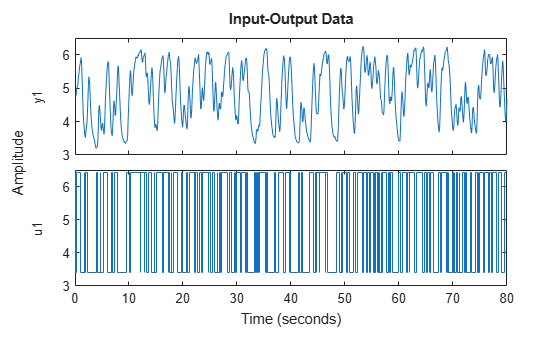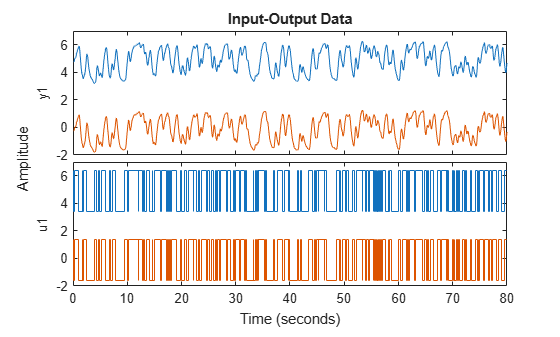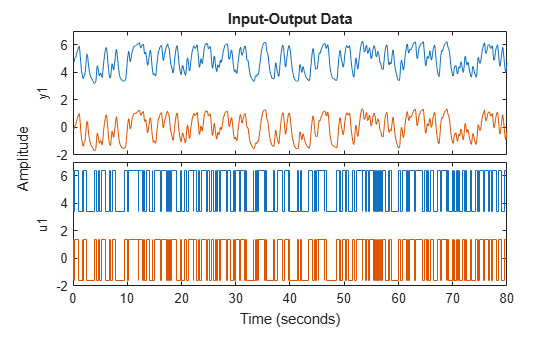getTrend

Create trend information object to store offset, mean, and trend information for time-domain signals stored in iddata object

Description

example

T = getTrend(data) constructs a TrendInfo object to store offset, mean, or linear trend information for detrending or retrending data. You can assign specific offset and slope values to T. You can then apply the trend information in T to either data or to other iddata objects by using detrend or retrend.

example

T = getTrend(data,0) computes the means of input and output signals and stores them as the InputOffset and OutputOffset properties of T, respectively.

example

T = getTrend(data,1) computes a best-fit straight line for both input and output signals and stores them as properties of T. The following code represents the straight line:

ULine = Tr.InputOffset + (time-t0)*Tr.InputSlope
YLine = Tr.OutputOffset + (time-t0)*Tr.OutputSlope
Here, time is equal to Z.SamplingInstants and t0 is equal to data.Tstart.

Examples

collapse all

Remove a specified offset from input and output signals.

Load SISO data containing vectors u2 and y2.

Create a data object with a sample time of 0.08 seconds and plot it.

data = iddata(y2,u2,0.08);
plot(data)The data has a nonzero mean value.

Store the data offset and trend information in a TrendInfo object.

T = getTrend(data);

Assign offset values to the TrendInfo object.

T.InputOffset = 5;
T.OutputOffset = 5;

Subtract the offsets from the data.

data_d = detrend(data,T);

Plot the detrended data on the same plot.

hold on
plot(data_d)View the mean value removed from the data.

get(T)
ans = struct with fields:
DataName: 'data'
InputOffset: 5
OutputOffset: 5
InputSlope: 0
OutputSlope: 0

Compute input-output signal means, store them, and detrend the data.

Load SISO data containing vectors u2 and y2.

Create a data object with a sample time of 0.08 seconds.

data = iddata(y2,u2,0.08);

Compute the mean of the data.

T = getTrend(data,0);

Remove the mean from the data.

data_d = detrend(data,T);

Plot the original and detrended data on the same plot.

plot(data,data_d)Load and plot data that contains two input channels and one output channel.

plot(z7L)The output channel of z7L contains a linear trend that is not present in the input channels. Compute the trend information.

T = getTrend(z7L,1)
Trend specifications for data "z7L" with 2 input(s), 1 output(s), 1 experiment(s):
DataName: 'z7L'
InputOffset: [-0.0764 -0.0683]
OutputOffset: -0.2642
InputSlope: [4.8338e-04 3.1642e-04]
OutputSlope: 0.0268

Limit the trend information to the output channel only by setting the input trend values to 0.

T.InputOffset = [0 0];
T.InputSlope = [0 0];
T
Trend specifications for data "z7L" with 2 input(s), 1 output(s), 1 experiment(s):
DataName: 'z7L'
InputOffset: [0 0]
OutputOffset: -0.2642
InputSlope: [0 0]
OutputSlope: 0.0268

Remove the linear trend from the data.

z7d = detrend(z7L,T);
plot(z7d)The trend is no longer in the output data and the input data is unchanged.

Input Arguments

collapse all

Time-domain input-output data, specified as an iddata object containing one or more sets of time-domain signals. The iddata object can contain SISO, MIMO, or multiexperiment data. The signal sets can contain either input and output data or output data only.

Output Arguments

collapse all

Trend information, returned as a TrendInfo object.